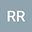Demonstration of a Technique to Construct a One-to-One Correspondence Between N and the Infinite Binary Decimals in (0,1)
•• Ron Ragusa
Ron Ragusa

Corresponding Author:ron.ragusa@gmail.com

Author Profile## Abstract

In this paper we will see how by varying the initial conditions of Cantor's Diagonal Argument we can use the method to produce a one-to-one correspondence between the set of natural numbers and the set of infinite binary decimals in the open interval (0, 1). We concede that the initial list of infinite binary decimals is, in fact, incomplete and that the diagonal method does produce a number not contained in the list. Also, we'll agree that there are an infinite number of binary decimal numbers in the interval that aren't in the list. We will see how using the same diagonal method we can create infinitely many binary decimal numbers not initially contained in the interval and that each number we so create will correspond with one and only one natural number.